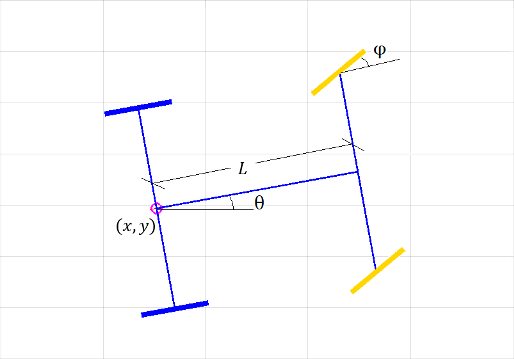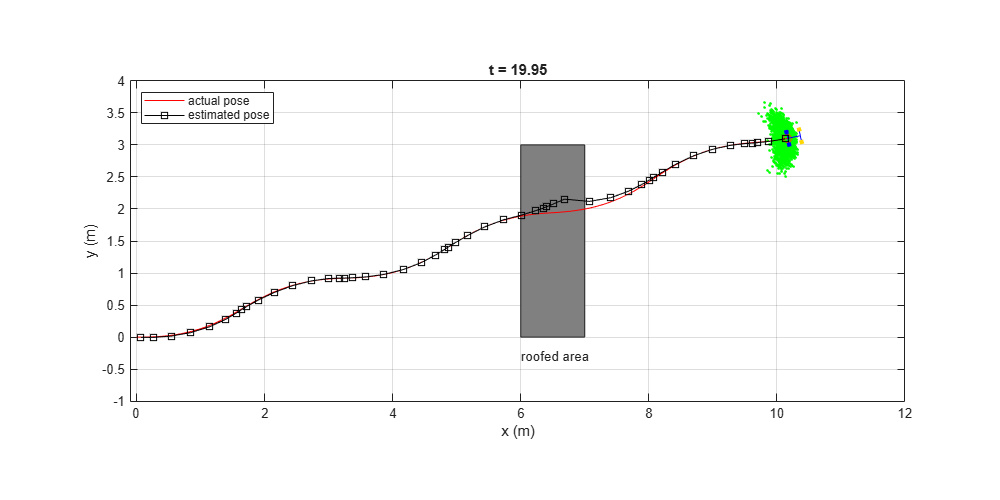# Track a Car-Like Robot Using Particle Filter

Particle filter is a sampling-based recursive Bayesian estimation algorithm, which is implemented in the `stateEstimatorPF` object. In this example, a remote-controlled car-like robot is being tracked in the outdoor environment. The robot pose measurement is provided by an on-board GPS, which is noisy. There are known motion commands sent to the robot, but the robot will not execute the exact commanded motion due to mechanical slack or model inaccuracy. This example will show how to use `stateEstimatorPF` to reduce the effects of noise in the measurement data and get a more accurate estimation of the pose of the robot. The kinematic model of a car-like robot is described by the following non-linear system. The particle filter is ideally suited for estimating the state of such kind of systems, as it can deal with the inherent non-linearities.

`$\begin{array}{cl}\underset{}{\overset{˙}{x}}& =v\mathrm{cos}\left(\theta \right)\\ \underset{}{\overset{˙}{y}}& =v\mathrm{sin}\left(\theta \right)\\ \underset{}{\overset{˙}{\theta }}& =\frac{v}{L}\mathrm{tan}\varphi \\ \underset{}{\overset{˙}{\varphi }}& =\omega \end{array}$`Scenario: The car-like robot drives and changes its velocity and steering angle continuously. The pose of the robot is measured by some noisy external system, e.g. a GPS or a Vicon system. Along the path it will drive through a roofed area where no measurement can be made.

Input:

• The noisy measurement on robot's partial pose ($x$, $y$, $\theta$). Note this is not a full state measurement. No measurement is available on the front wheel orientation ($\varphi$) as well as all the velocities ($\underset{}{\overset{˙}{x}}$, $\underset{}{\overset{˙}{y}}$, $\underset{}{\overset{˙}{\theta }}$, $\underset{}{\overset{˙}{\varphi }}$).

• The linear and angular velocity command sent to the robot (${v}_{c}$, ${\omega }_{c}$). Note there will be some difference between the commanded motion and the actual motion of the robot.

Goal: Estimate the partial pose ($x$, $y$, $\theta$) of the car-like robot. Note again that the wheel orientation ($\varphi$) is not included in the estimation. From the observer's perspective, the full state of the car is only [ $x$, $y$, $\theta$, $\underset{}{\overset{˙}{x}}$, $\underset{}{\overset{˙}{y}}$, $\underset{}{\overset{˙}{\theta }}$ ].

Approach: Use `stateEstimatorPF` to process the two noisy inputs (neither of the inputs is reliable by itself) and make best estimation of current (partial) pose.

• At the predict stage, we update the states of the particles with a simplified, unicycle-like robot model, as shown below. Note that the system model used for state estimation is not an exact representation of the actual system. This is acceptable, as long as the model difference is well-captured in the system noise (as represented by the particle swarm). For more details, see `predict`.

`$\begin{array}{cl}\underset{}{\overset{˙}{x}}& =v\mathrm{cos}\left(\theta \right)\\ \underset{}{\overset{˙}{y}}& =v\mathrm{sin}\left(\theta \right)\\ \underset{}{\overset{˙}{\theta }}& =\omega \end{array}$`

• At the correct stage, the importance weight (likelihood) of a particle is determined by its error norm from current measurement ($\sqrt{\left(\Delta x{\right)}^{2}+\left(\Delta y{\right)}^{2}+\left(\Delta \theta {\right)}^{2}}$), as we only have measurement on these three components. For more details, see `correct`.

### Initialize a Car-like Robot

```rng('default'); % for repeatable result dt = 0.05; % time step initialPose = [0 0 0 0]'; carbot = ExampleHelperCarBot(initialPose, dt);```### Set up the Particle Filter

This section configures the particle filter using 5000 particles. Initially all particles are randomly picked from a normal distribution with mean at initial state and unit covariance. Each particle contains 6 state variables ($x$, $y$, $\theta$, $\underset{}{\overset{˙}{x}}$, $\underset{}{\overset{˙}{y}}$, $\underset{}{\overset{˙}{\theta }}$). Note that the third variable is marked as Circular since it is the car orientation. It is also very important to specify two callback functions `StateTransitionFcn` and `MeasurementLikelihoodFcn`. These two functions directly determine the performance of the particle filter. The details of these two functions can be found the in the last two sections of this example.

```pf = stateEstimatorPF; initialize(pf, 5000, [initialPose(1:3)', 0, 0, 0], eye(6), 'CircularVariables',[0 0 1 0 0 0]); pf.StateEstimationMethod = 'mean'; pf.ResamplingMethod = 'systematic'; % StateTransitionFcn defines how particles evolve without measurement pf.StateTransitionFcn = @exampleHelperCarBotStateTransition; % MeasurementLikelihoodFcn defines how measurement affect the our estimation pf.MeasurementLikelihoodFcn = @exampleHelperCarBotMeasurementLikelihood; % Last best estimation for x, y and theta lastBestGuess = [0 0 0];```

### Main Loop

Note in this example, the commanded linear and angular velocities to the robot are arbitrarily-picked time-dependent functions. Also, note the fixed-rate timing of the loop is realized through `rateControl`.

Run loop at 20 Hz for 20 seconds using fixed-rate support.

`r = rateControl(1/dt);`

Reset the fixed-rate object to restart the timer. Reset the timer right before running the time-dependent code.

```reset(r); simulationTime = 0; while simulationTime < 20 % if time is not up % Generate motion command that is to be sent to the robot % NOTE there will be some discrepancy between the commanded motion and the % motion actually executed by the robot. uCmd(1) = 0.7*abs(sin(simulationTime)) + 0.1; % linear velocity uCmd(2) = 0.08*cos(simulationTime); % angular velocity drive(carbot, uCmd); % Predict the carbot pose based on the motion model [statePred, covPred] = predict(pf, dt, uCmd); % Get GPS reading measurement = exampleHelperCarBotGetGPSReading(carbot); % If measurement is available, then call correct, otherwise just use % predicted result if ~isempty(measurement) [stateCorrected, covCorrected] = correct(pf, measurement'); else stateCorrected = statePred; covCorrected = covPred; end lastBestGuess = stateCorrected(1:3); % Update plot if ~isempty(get(groot,'CurrentFigure')) % if figure is not prematurely killed updatePlot(carbot, pf, lastBestGuess, simulationTime); else break end waitfor(r); % Update simulation time simulationTime = simulationTime + dt; end```### Details of the Result Figures

The three figures show the tracking performance of the particle filter.

• In the first figure, the particle filter is tracking the car well as it drives away from the initial pose.

• In the second figure, the robot drives into the roofed area, where no measurement can be made, and the particles only evolve based on prediction model (marked with orange color). You can see the particles gradually form a horseshoe-like front, and the estimated pose gradually deviates from the actual one.

• In the third figure, the robot has driven out of the roofed area. With new measurements, the estimated pose gradually converges back to the actual pose.

### State Transition Function

The sampling-based state transition function evolves the particles based on a prescribed motion model so that the particles will form a representation of the proposal distribution. Below is an example of a state transition function based on the velocity motion model of a unicycle-like robot. For more details about this motion model, please see Chapter 5 in . Decrease `sd1`, `sd2` and `sd3` to see how the tracking performance deteriorates. Here `sd1` represents the uncertainty in the linear velocity, `sd2` represents the uncertainty in the angular velocity. `sd3` is an additional perturbation on the orientation.

``` function predictParticles = exampleHelperCarBotStateTransition(pf, prevParticles, dT, u) ```
``` thetas = prevParticles(:,3); ```
``` w = u(2); v = u(1); ```
``` l = length(prevParticles); ```
``` % Generate velocity samples sd1 = 0.3; sd2 = 1.5; sd3 = 0.02; vh = v + (sd1)^2*randn(l,1); wh = w + (sd2)^2*randn(l,1); gamma = (sd3)^2*randn(l,1); ```
``` % Add a small number to prevent div/0 error wh(abs(wh)<1e-19) = 1e-19; ```
``` % Convert velocity samples to pose samples predictParticles(:,1) = prevParticles(:,1) - (vh./wh).*sin(thetas) + (vh./wh).*sin(thetas + wh*dT); predictParticles(:,2) = prevParticles(:,2) + (vh./wh).*cos(thetas) - (vh./wh).*cos(thetas + wh*dT); predictParticles(:,3) = prevParticles(:,3) + wh*dT + gamma*dT; predictParticles(:,4) = (- (vh./wh).*sin(thetas) + (vh./wh).*sin(thetas + wh*dT))/dT; predictParticles(:,5) = ( (vh./wh).*cos(thetas) - (vh./wh).*cos(thetas + wh*dT))/dT; predictParticles(:,6) = wh + gamma; ```
``` end ```

### Measurement Likelihood Function

The measurement likelihood function computes the likelihood for each predicted particle based on the error norm between particle and the measurement. The importance weight for each particle will be assigned based on the computed likelihood. In this particular example, `predictParticles` is a N x 6 matrix (N is the number of particles), and `measurement` is a 1 x 3 vector.

``` function likelihood = exampleHelperCarBotMeasurementLikelihood(pf, predictParticles, measurement) ```
``` % The measurement contains all state variables predictMeasurement = predictParticles; ```
``` % Calculate observed error between predicted and actual measurement % NOTE in this example, we don't have full state observation, but only % the measurement of current pose, therefore the measurementErrorNorm % is only based on the pose error. measurementError = bsxfun(@minus, predictMeasurement(:,1:3), measurement); measurementErrorNorm = sqrt(sum(measurementError.^2, 2)); ```
``` % Normal-distributed noise of measurement % Assuming measurements on all three pose components have the same error distribution measurementNoise = eye(3); ```
``` % Convert error norms into likelihood measure. % Evaluate the PDF of the multivariate normal distribution likelihood = 1/sqrt((2*pi).^3 * det(measurementNoise)) * exp(-0.5 * measurementErrorNorm); ```
``` end ```

### Reference

 S. Thrun, W. Burgard, D. Fox, Probabilistic Robotics, MIT Press, 2006